# Swift Program to sort an array in ascending order using insertion sort

In Swift, insertion sort is a sorting technique in which the given array is virtually divided into two parts that are the sorted and the unsorted part. Then the array is searched sequentially, compares two elements from the unsorted part, and moves them into the right place in the sorted part. Using insertion sort, we can easily sort the array elements in ascending or descending order. So in this article, we will learn how to sort an array in ascending order using insertion sort.

## Working of insertion sort

Given unsorted array −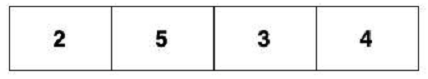To sort the array in ascending order, compare the first two elements which are 2 and 5.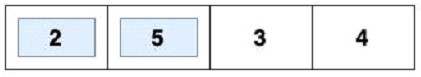Here both 2 and 5 are in ascending order. So we move to the next two elements which are 5 and 3.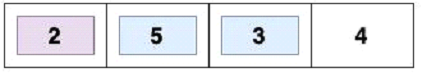Here 5 is greater than 3 so we swap the position of 5 and 3.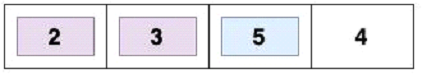Now we again compare 2 and 3 here both 2 and 3 are in ascending order so we move to the next elements which are 5 and 4.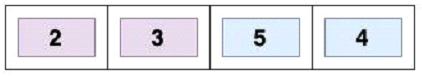Here 5 is greater than 4 so we swap the positions of the elements.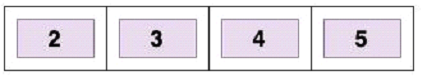Again compare elements 3 and 4, here both the elements are in ascending order. Hence we get the final sorted array in ascending order. So this is how insertion sort works.

## Algorithm

• Step 1 − Create a function which takes an array as an argument and returns the sorted array.

• Step 2 − Inside the function create a copy of the original array.

• Step 3 − Iterate a for-in loop through each array element starting from index 1 to n-1.

• Step 4 − Then run a while loop and compare the current element to its predecessor element.

• Step 5 − If the element in the sorted array is smaller than the current element, move to the next element. If not then shift the greater element to the right.

• Step 6 − Insert the current value in the resultant array.

• Step 7 − Return the sorted array.

• Step 8 − Create an array, call the function, and pass it into it.

• Step 9 − Print the output.

## Example

In the following Swift program, we will sort an array in ascending order using insertion sort. So for that, we will create an array and then we will pass that array in the function. Then the function creates a copy of the original array. Then it iterates over the elements of the array starting from index 1 and for each element it will store its value in the initalVal. Then it starts a while loop which moves each element that is greater than initialVal one position to the right. This loop continues till the elements present in the initialVal are greater than initialVal.After that, the function inserts initialVal in the sorted array at the correct position and finally will return the sorted array.

import Foundation
import Glibc

func sorting(arr:[Int]) -> [Int] {
var resultantArray = arr

for x in 1..<resultantArray.count{
let initialVal = resultantArray[x]
var y = x

// Moving elements that are greater than initialVal
// one position on the right
while y > 0 && resultantArray[y-1] > initialVal{
resultantArray[y] = resultantArray[y-1]
y -= 1
}
resultantArray[y] = initialVal
}
return resultantArray
}

var mArr = [3, 1, 9, 4, 2, 10, 5]
var res = sorting(arr:mArr)

print(res)


## Output

[1, 2, 3, 4, 5, 9, 10]


## Conclusion

So this is how we can sort an array in ascending order using insertion sort. Insertion sort is the easiest sorting algorithm, but it only works for a small data set. It does not work well with larger data sets. The worst-case time complexity of the insertion sort is O(N2), and the rest time complexity is O(N)

Updated on: 10-May-2023

189 Views